## Altitude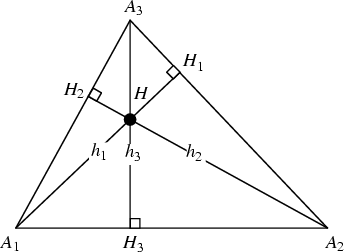The altitudes of a Triangle are the Cevianswhich are Perpendicular to the Legsopposite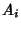. They have lengths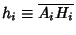given by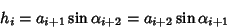(1)(2)

whereis the Semiperimeter of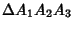and. Another interesting Formula is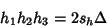(3)

(Johnson 1929, p. 191), whereis the Area of the Triangleandis the Semiperimeter of the altitude triangle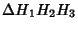. The three altitudes of any Triangle are Concurrent at the Orthocenter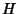. This fundamental fact did not appear anywhere in Euclid'sElements.

Other formulas satisfied by the altitude include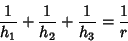(4)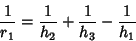(5)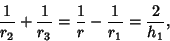(6)

whereis the Inradius andare the Exradii (Johnson 1929, p. 189). In addition,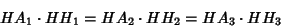(7)(8)

whereis the Circumradius.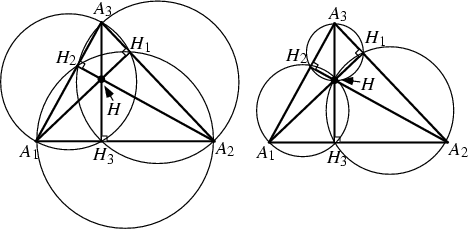The points,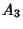,, and(and their permutations with respect to indices) all lie on a Circle, as do the points,,, and(and their permutations with respect to indices). Trianglesand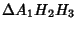are inversely similar.

The trianglehas the minimum Perimeter of any Triangle inscribed in a given Acute Triangle (Johnson 1929, pp. 161-165). The Perimeter ofis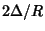(Johnson 1929, p. 191). Additional properties involving the Feet of the altitudes are given by Johnson (1929, pp. 261-262).